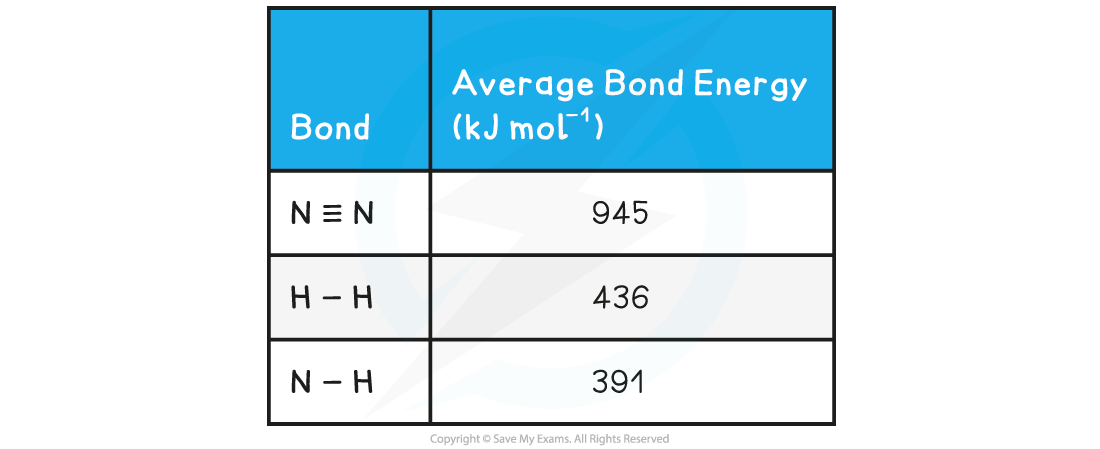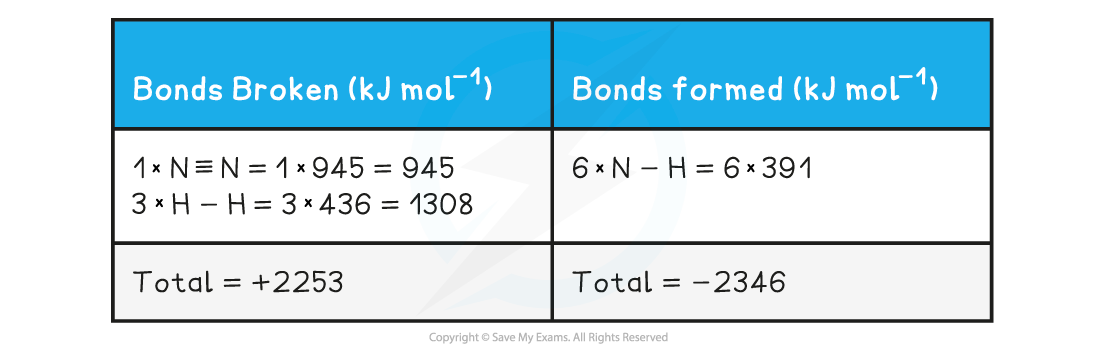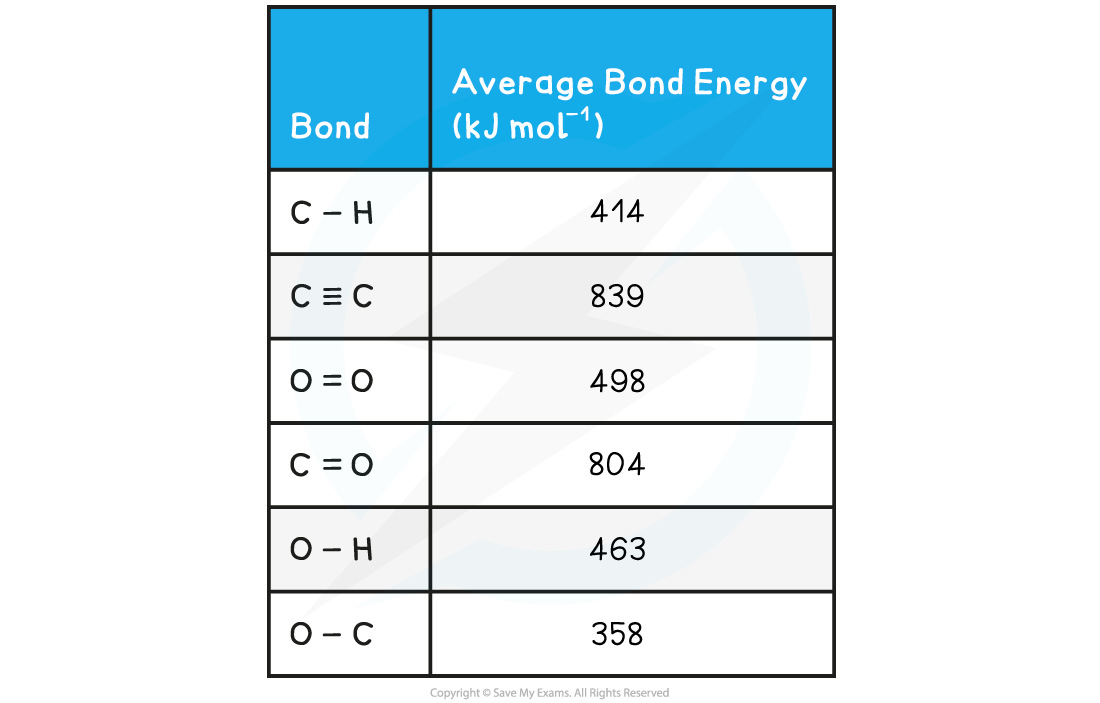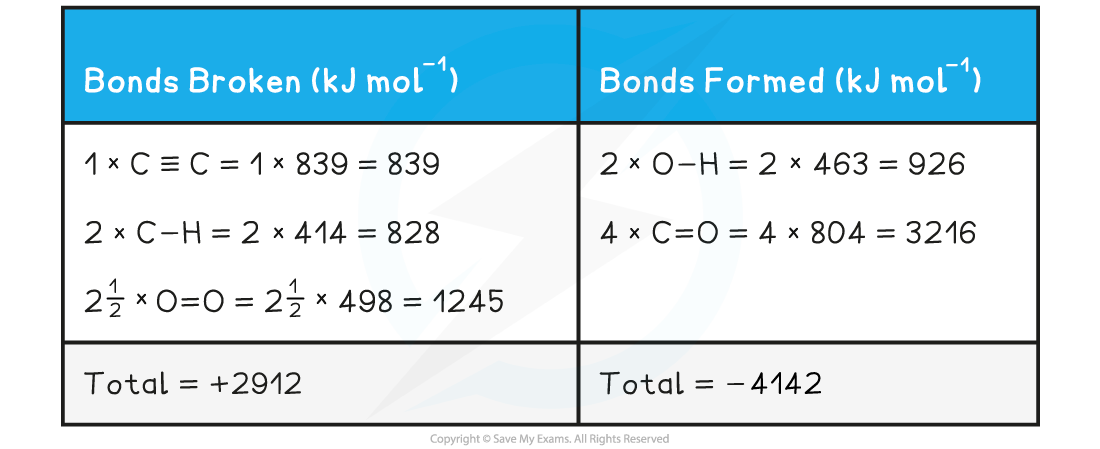# IB DP Chemistry: SL复习笔记5.3.2 Bond Enthalpy Calculations

### Bond Enthalpy Calculations

• Bond energies are used to find the ΔHr of a reaction when this cannot be done experimentally
• The process is a step-by-step summation of the bond enthalpies of the all the molecules present finishing with this formula:Formula for calculating the standard enthalpy change of reaction using bond energies

• These two worked examples show how to lay out your calculation

#### Worked Example

Calculate the enthalpy of reaction for the Haber process reaction. The relevant bond energies are given in the table below:Step 1: The chemical equation for the Haber process is:

N2 (g) + 3H2 (g) ⇌ 2NH3 (g)

N≡N       3 H-H         6 N-H

Step 2: Set out the calculation as a balance sheet as shown below:Note! Values for bonds broken are positive (endothermic) and values for bonds formed are negative (exothermic)

Step 3: Calculate the standard enthalpy of reaction

ΔHr = enthalpy change for bonds broken + enthalpy change for bonds formed

= (+2253 kJ mol-1) + (-2346 kJ mol-1)

= -93 kJ mol-1

#### Worked Example

The complete combustion of ethyne, C2H, is shown in the equation below:

2C2H2 (g)  + 5 O2 (g)      2H2O (g) + 4CO2 (g)

Using the average bond enthalpies given in the table, what is the enthalpy of combustion of ethyne?Step 1: The enthalpy of combustion is the enthalpy change when one mole of a substance reacts in excess oxygen to produce water and carbon dioxide

The chemical reaction should be therefore simplified such that only one mole of ethyne reacts in excess oxygen:

H-C≡C-H + 2 ½ O=O → H-O-H + 2O=C=O

Step 2: Set out the calculation as a balance sheet as shown below:ΔHr = enthalpy change for bonds broken + enthalpy change for bonds formed

= (+2912 kJ mol-1) + (- 4142 kJ mol-1)

= -1230 kJ mol-1

#### Exam Tip

The key to success in bond enthalpy calculations is to be very careful when accounting for every bond present. Always draw out the full displayed structures of the molecules so you don't miss any of the bonds.

Watch out for coefficients in the balanced equations as students often miss those, forget to multiply them by the bond enthalpies and get the answer wrong!

It is super important to show your steps because bond enthalpy calculations often carry 3 marks, 2 of which could be for workings if you get the final answer wrong